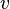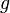## Posts Tagged ‘maths’

### Seventeen equations that changed the world

March 20, 2014

I just came across this summarising Ian Stewart’s book on 17 Equations That Changed The World at Business Insider:

I have used all of these up to Equation 12. I have never used the equations on Relativity or Schrodinger’s equation or those on Chaos or Information theory or the Black-Scholes Equation. But, I wouldn’t disagree with Equations 12 – 17, but considering the amount of time I spent applying it at University and during my working life I would have liked to see Bernoulli’s Equation on the list:where:is the fluid flow speed at a point on a streamline,is the acceleration due to gravity,is the elevation of the point above a reference plane, with the positive z-direction pointing upward – so in the direction opposite to the gravitational acceleration,is the pressure at the chosen point, andis the density of the fluid at all points in the fluid.

June 16, 2013

I like this one:

## π = 3For the error in this one and some other similar math errors go HERE.